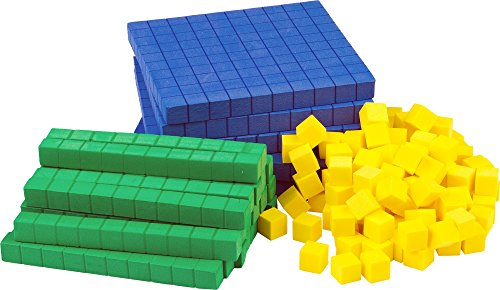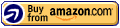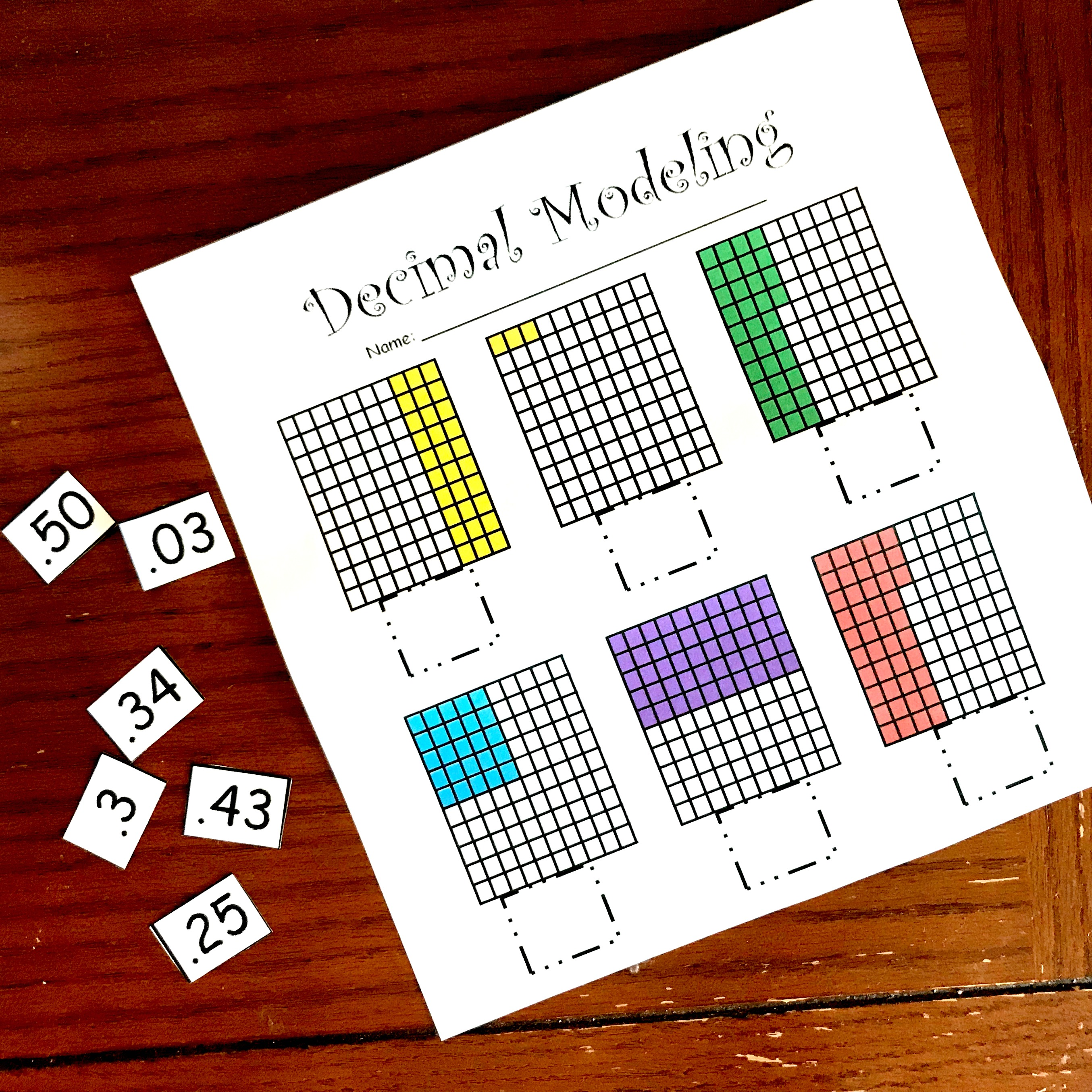# 3 Hands-On Decimal Place Value Worksheets

Are you introducing decimals? Then these decimal place value worksheets are a fun way to practice decimals on the number line, through modeling decimals, and the value of decimals compared to 1/2.

### The free printable can be found at the END of this post. Click on the blue “get your free printable here” button.

In the younger grades, we focus a lot on place value. We want them to understand what each digit stands for and we practice using 100’s charts, expanded form, and base ten blocks. As children move into upper grades, the focus switches to fractions and decimals…..and it is just as important to focus on place value here.

Today I have three decimal place value worksheets to help children learn and understand about decimals and the value of the digits in the tenths place and thousandths place.

Get all my Decimal Printables HERE!!!

## Prep-Work For Decimal Place Value Worksheets

These worksheets are no prep sheets.

1. First print off the sheets.
2. Then provide glue and scissors.

And you are ready to go.

## Decimal Modeling

The first cut and paste sheet allows children to focus on modeling decimals. The children are provided grids with 100 squares, and parts of the grid are colored in.

Before having the children work through this cut and paste decimal activity, children must understand decimal place value.

Knowing that the first digit to the right of a decimal represents a tenth is essential. And they must also recognize that when one full line in a 100’s grid is colored in it equals a tenth. (One out of 10 lines are colored in)

In this example, you can see that three lines are colored in, and therefore this grid equals 3/10, three-tenths, or .3. Or you could say that it equals 30/100, thirty-hundredths, or .30. They are all the same number!

In this example, you can see that only three little squares are colored in. Using our knowledge of fractions we can see that 3 out of 100 are colored in. So my decimal looks something like this. I have zero tenths, so a 0 goes in the tenths spot and a 3 in the hundredths spot….. (0.03)

You can practice this concept more with a decimal game.

## Decimals on a Number Line

Number lines are a great way to build number sense in children, and they can also help children develop decimal place value sense too.

The number lines shown in this example have 10 lines. Each line represents one-tenth and helps children build the concept that a decimal is between 0 and 1.

To complete this sheet, the children just need to place the decimals on the number line in the correct spot.

1. First, they will cut out the numbers.
2. Next, encourage the children to count up the number line and then find the number that goes in the box.

For example, a child might say…..

zero, one-tenth, two-tenths and then find the number that shows .2.

You can get in more practice with this free number line game.

## Using Place Value to Compare Fractions

Base ten blocks are a fantastic way to help children conquer this final decimal place value worksheet.Teacher Created Resources Foam Base Ten Set (20617)Begin by stating that a flat equals one whole, and then have the children figure out what a long stands for and what the cube stands for.

They should be able to tell you that a since it takes 10 longs to fill up a flat that they equal one-tenth (0.1). And since you will need 100 cubes to fill up a flat, a cube equal one-hundredth (0.01).

Next, look at what number we will be comparing with. In this worksheet, they are comparing to 1/2. So the children should take out 5 longs….which equals half of a flat…..And I wouldn’t tell my kiddos this, I would let them figure it out on their own.

Now they look at each number provided and create that number with base ten blocks. The first number is .31. So they should take out three longs and one cube. When they put this next to the five longs they can easily see that it is less than 1/2.

Therefore .31 goes in the column that says less than 1/2.

Children learn place value by exploring and I hope these sheets help them explore decimals in a fun way!!

You’ve Got This

Rachel

You May Also Like:

Decimal of the Day

The free sample has three cut and paste sheets, but you can get all 10 HERE.

You’ll find it under the decimal section!